# Quantifying robustness of biochemical network models

## Abstract

### Background

Robustness of mathematical models of biochemical networks is important for validation purposes and can be used as a means of selecting between different competing models. Tools for quantifying parametric robustness are needed.

### Results

Two techniques for describing quantitatively the robustness of an oscillatory model were presented and contrasted. Single-parameter bifurcation analysis was used to evaluate the stability robustness of the limit cycle oscillation as well as the frequency and amplitude of oscillations. A tool from control engineering – the structural singular value (SSV) – was used to quantify robust stability of the limit cycle. Using SSV analysis, we find very poor robustness when the model's parameters are allowed to vary.

### Conclusion

The results show the usefulness of incorporating SSV analysis to single parameter sensitivity analysis to quantify robustness.

## Background

Complex molecular networks mediate intracellular signalling events. These systems must operate reliably under vastly different environmental conditions that can cause large changes in the internal "parameters" of the system. The notion of robustness in biological systems has received considerable interest in the literature recently. By saying that a system is robust we imply that a particular function or characteristic of the system is preserved despite changes in the operating environment of the system. For example, by means of a computer model, Barkai and Leibler demonstrated that the adaptation mechanism found in the chemotactic signalling pathway in Escherichia coli is robust . This was later confirmed experimentally . A model of segment polarity network in Drosophila embryos was also found to be insensitive to variations in kinetic constants that govern its behaviour . A similar approach was later used to show that a core neurogenic network in Drosophila successfully formed three test patterns across a wide range of parameter values  leading Meir et al. to propose that the ability to resist parameter fluctuations may be essential for gene network evolutionary flexibility.

Since the signalling pathways are robust, we should expect that mathematical models that attempt to explain these networks also be robust to parameter variations. This has long been appreciated. For example, Savageau, in , argues for parameter sensitivities as a means of evaluating the performance of biochemical systems. More recently, Morohashi et al. propose that robustness of a model to parameter variations be used as a criterion for determining plausibility between different models .

If we are to use robustness as a means of evaluating the quality of a model, we need objective measures of this robustness. One common technique is through parameter sensitivities. For simple systems, the sensitivity of a model of a network to individual parameters can be evaluated analytically [5, 7]. For more complex networks, it can be determined computationally by repeated simulation varying one parameter while holding all others fixed; [3, 8]. This single parameter sensitivity is also useful for testing robustness of a biochemical network in the laboratory. For example, it is by systematically varying the concentration of the chemotaxis-network proteins in E. coli and determining their effect – or lack thereof – on the precision of adaptation that Alon et al. determined the robustness of this system .

Single parameter insensitivity is necessary for a robust network, but may not be sufficient owing to interactions between several parameters. This is particularly true in vivo where many different system parameters will differ from their "nominal" values simultaneously. The tools available for quantifying this multiparametric uncertainty are more limited. Systematic changes of many parameters at a time suffer from an exponential increase in the number of parameters that need to be changed. This "curse of dimensionality" makes varying more than a handful of parameters simultaneously to assess parameter sensitivity impractical. For this reason, sensitivities for several parameters have been traditionally addressed through computer simulations based on Monte Carlo methods  – randomly varying all parameter in the model [1, 4]. However, because of their reliance on random methods, Monte Carlo methods cannot guarantee robustness. In this paper we suggest an alternative method, originally developed for use in analysing robust stability in man-made automatic control systems.

The need for robust systems has been one of the primary concerns of control engineering. In fact, one of the earliest motivations for the study of feedback control systems was the need to create robust telephone networks out of the highly variable vacuum tubes of the day. More recently, powerful tools for analysing the robustness of networks have emerged. In this paper we propose that one of these computational tools, known in control theory as the structural singular value (SSV) is of particular interest for biological networks . We do this by contrasting single and multi-parameter sensitivities of a model of an oscillating biochemical network. We describe this model next.

### Model of an oscillating biochemical network

In , Laub and Loomis propose a model of the molecular network underlying adenosine 3',5'-cyclic monophosphate (cAMP) oscillations observed in fields of chemotactic Dictyostelium discoideum cells. The model, based on the network depicted in Fig. 1, induces the spontaneous oscillations in cAMP observed during the early development of D. discoideum.

In this model, changes in the enzymatic activities of these proteins are described by a system of seven non-linear differential equations: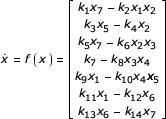where the state variable x = [x1,...,x7] represents the concentrations of the seven proteins: x1 = [ACA], x2 = [PKA], x3 = [ERK2], x4 = [REG A], x5 = [Internal cAMP], x6 = [External cAMP] and x7 = [CAR1] and the fourteen different k i represent system parameter values. It was shown numerically in  that spontaneous oscillations appear at the nominal parameter values found in Table 1. Note that because there are typographical errors in the original paper, the values being used here for the nominal parameters were obtained directly from the authors of .

This particular model is primarily concerned with describing self-sustaining oscillations in the biochemical system. From Fig. 2, it is clear that at the nominal parameter values of the model, this is achieved. We seek to determine whether the system is robust – that is, if we change these kinetic parameters, will the systems oscillatory behaviour persist? We next present two possible means, based on whether parameters are changed one at a time or in groups.

## Methods

### Single parameter robustness: Bifurcation analysis

Self-sustained oscillations such as those being modelled here appear as stable limit cycles in trajectory of the underlying dynamical system . The existence and stability of these limit cycles may change under parametric perturbations. Whenever a stable periodic solution loses stability as we vary the underlying parameters of the system and this solution transitions to another qualitative solution – for example, a steady-state equilibrium – we say that the system undergoes a Hopf bifurcation. It is therefore possible to use bifurcation theory as a means of quantifying the robustness of this oscillatory network model [12, 13].

Using the bifurcation analysis package AUTO , it is possible to produce one-parameter bifurcation diagrams for each of the model parameters k i . These diagrams illustrate the steady-state behaviour of the systems as the parameter values are changed. Suppose that Hopf bifurcations occur at k i and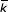i so that (stable) limit cycles occur for the range (k i ,i ). Both the size of this interval as well as the proximity of the nominal parameter value to either boundary are measures of the robustness of the system. To compare the robustness of the system to the different parameters, we can define a series of parametric robustness measures. We define the degree of robustness (DOR) for each parameter k i as: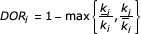It is straightforward to see that this value is always between zero and one. The former indicates extreme parameter sensitivity whereas the latter implies large insensitivity.

Bifurcation diagrams provide an excellent means of determining the robustness of systems to single parameter perturbations. We next describe a method for analysing and quantifying robustness to simultaneous changes in several parameters.

### Multiparametric robustness: Structural singular values

As in biological networks, engineering systems must also be robust to variations in the parametric values of its components. Developing tools for the analysis and design of robust automatic control systems has been an area of active research during the last two decades in control theory. One of the most powerful frameworks for measuring robustness known is the structural singular value (SSV) which is due to Doyle and co-workers .

We first define and illustrate the use of the SSV to quantify robustness by means of a simple example. Suppose that a system is described by the first-order differential equation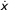= ax

where the constant parameter a is uncertain, but is assumed to lie in the region a (a,).

We would like to know when this system is robustly stable; that is, it is stable for all possible parameters. The differential equation can be rewritten as= a0x + b0v     (1)

where a0 = (+ a)/2, b0 = (- a)/2, v = δx and δ (-1,1). In Eqn. (1), the term a0 represents the nominal system description. Clearly, for the system to be stable we need a0 < 0. The variable δ represents all possible uncertainty in the parameter of the system, whereas b0 dictates the uncertainty's effect on the nominal model. We would like to maintain system stability no matter what the value of δ happens to be. Note that the system can be redrawn in the form of a feedback loop as in Fig. 3A. From the small gain theorem , it is known that if the nominal system is stable (a0 < 0), the uncertain system will remain so whenever the gain around the loop is less than one. That is, if we denote the system transfer function – the ratio of Fourier transforms of output over input – as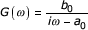(here i refers to the complex number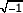and ω is the angular frequency) then the system is stable provided that 1 - G(ω)δ ≠ 0, for all frequencies ω. Equivalently, the amount of uncertainty that the system can tolerate is given by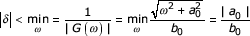Thus, the function μ = |G(ω)| serves as a (frequency-dependent) measure of the amount of parameter uncertainty that the system can tolerate. In particular, if the size of the uncertainty δ is always less than 1/μ, then the system is robustly stable. In this example, since |δ| < 1, robust stability is guaranteed whenever b0 < |a0|.

It is clear that this simple model does not require extensive analytic tools to determine robust stability. Nevertheless, the procedure above can be generalized to systems of the form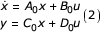where A0, is a matrix describing the nominal model, B0, C0, and D0 are matrices of appropriate dimensions describing the way that the uncertain parameters affect the nominal model. This uncertainty is modelled by the matrix Δ which is unknown, but is assumed to belong to the set. The signal u = Δy completes the feedback loop as shown in Fig. 3B. For these systems, the appropriate measure of robustness is now given by the structural singular value (SSV)

μΔ (G) = (min{||Δ||:Δ, det(I - G Δ) ≠ 0})-1     (3)

Here, G(ω) = C0 (iωI - A0)-1 B0 + D0 and I is the identity matrix of appropriate dimensions.

Since we are interested in the robustness of the oscillatory property of this system, it is natural to use the SSV to quantify the robust stability of the limit cycle. However, in order to use the SSV tool, the original perturbed system must be transformed into a framework consisting of a nominal linear time invariant (LTI) system interconnected with a perturbation matrix. For the case of an oscillatory non-linear model, this involves several steps, which we outline next.

#### Determining the limit cycle: Harmonic balance method

The first step is to obtain a mathematical expression for the limit cycle oscillation. The harmonic balance method can be used . The basic idea is to represent the limit cycle by a Fourier series with unknown coefficients (an,i, φn,i) and period T: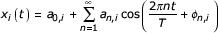The non-linear differential equation can be used to set up a series of algebraic equations that the coefficients must satisfy. These equations can be solved using numerical packages such as Mathematica or Maple. Depending on the particular form of the limit cycle, a small finite number of coefficients can be used. We can denote this periodic solution as x*(t).

#### Linearization

The non-linear differential equation must now be linearized about this periodic orbit . Writing the state vector x(t) as

x(t) = x*(t) + xδ (t)

then the local behaviour of the non-linear system is governed by that of the linearized system:δ (t) J (x*(t))x (t)

where J is the Jacobian matrix of the vector field f. Note that since the linearization is performed about a periodic orbit, the linear system is periodic.

#### Restructuring into nominal/uncertainty systems

The Jacobian matrix includes all uncertain parameters. At this point we need to separate the system into a nominal model and a feedback interconnection that involves all parametric uncertainty. We first write each parameter as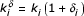where k i is the nominal value and δ i is the relative amount of perturbation in the ith parameter. We now separate the Jacobian matrix as

J (x*(t)) = A0 (t) + B0 (t) Δ C0 (t)     (4)

where A0(t) is the Jacobian matrix with all parameters at their nominal value, and Δ is a diagonal matrix containing all the uncertainties δ i . Let y(t) = C0(t) xδ(t) and u(t) = Δy(t), the system is now of the form of Eqn. (2) (with x(t) replaced by xδ(t)).

#### Discretization

The system can be discretized by sampling the state and output with sampling period h = T/n, where n is a positive integer and assuming that the inputs are piecewise constant; this is also a standard technique in control engineering . In particular, a linear continuous-time system governed by Eqn. (2) gives rise to the discrete-time, linear system

ξ (k + 1) = A d (k) ξ(k) + B d (k) v(k)

η (k) = C d (k) ξ(k)

where A d (k) = Φ (kh + h,kh), B d (k) =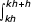Φ (kh + h, s) B0 (s)ds, C d (k) = C0 (kh), and Φ (t, τ) is the transition matrix of A0 (t) . The discretized signals are v(k) = u(kh), η(k) = y(kh), and ξ(k) = x(kh). Periodicity of A d and B d is preserved due to the periodicity of the transition matrix. Moreover, it is not difficult to confirm that A d , B d and C d are periodic with period n. The uncertainty matrix after discretization is now Δ d . The discretization step is illustrated in Fig. 4A.

#### Lifting

The final step in preparing the system for SSV analysis is to transform the periodic, linearized system into an equivalent time-invariant one. The technique for this is known as lifting . Rather than giving the general formulae, it is easier to illustrate the general principle with an example.

Suppose that a discrete-time system with state variable ξ, input v, and output η obeys the difference equation

ξ (k + 1) = a(k)ξ(k) + b(k)v(k)

η (k) = c(k)ξ(k)

where the time varying coefficients a(k), b(k) and c(k) are all periodic with period two. Calculating the state variable and output step-by-step leads to: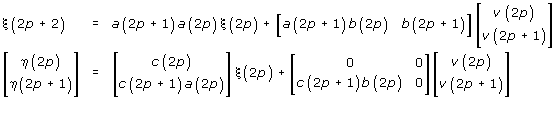for any integer p. By defining "lifted" inputs and outputsand considering the system state only at the even time points ((p) = ξ(2p)) we arrive at an equivalent time-invariant system.

The lifting technique has been illustrated above for a discrete-time system with period two; however, it can be applied to systems with arbitrary period – though the corresponding formulae are considerably more complicated; see .

#### Computation of SSV

There is considerable literature in control theory on the computation of the SSV; see for example . For general classes of uncertainty, computing μΔ is known to be NP-hard . Typically, given the feedback loop consisting of G and Δ we compute upper and lower bounds for the SSV . The lower bound is exactly equal to μΔ ; unfortunately computing this lower bound involves a search over a non-convex set and therefore may converge to local optimums that are not global. In contrast, the upper bound can be rewritten in terms of a convex optimisation problem, so that a global minimum can be obtained. However, this upper bound is, in general not tight. A software package is commercially available that can compute μupperand uses a power algorithm to attempt to compute μlower.

## Results

### Single parameter robustness

The robustness of Laub and Loomis's oscillatory model was first analysed by means of single-parameter bifurcation diagrams. Four typical diagrams are shown in Fig. 5. The activity of internal cAMP (x5) is plotted as a function of the variation of each parameter. We use internal cAMP in the diagram as it is the element that is usually observed experimentally . In each of the diagrams, there are three types of solutions: stable steady state, unstable steady state and limit cycle oscillations.

These diagrams illustrate that Hopf bifurcations occur for each parameter; that is, the oscillatory behaviour exists only in a limited range of parameters around the nominal value. For each of these parameters, the respective intervals and values for degree-of-robustness are found in Table 1.

### Structural singular value

From the numerical simulation (Fig. 2) of the non-linear model, we observed that the oscillatory curves did not deviate greatly from a simple harmonic oscillator plus a constant offset. Thus, to obtain an analytic expression for the periodic orbits we assume that the state variables are expanded into Fourier series containing only the fundamental and constant terms: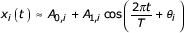for each of the seven states. Since it is the relative phase shift between each state variable that is relevant, we assume that θ1 = 0. The substitution of the Fourier series into the original equations leads to a series of real algebraic equations for the coefficients (not shown) whose solution was obtained using Mathematica. This leads us to obtain the corresponding periodic solutions where the values of A0,i, A1,iand θ i are found in Table 2. The period T is approximately 7.31 minutes. This analytic solution matches well with the numerical simulation except for an arbitrary phase shift, which does not affect the shape and location of the limit cycle in the phase space and can thereby be ignored (not shown).

Following our prescribed methods, we next linearized the system. The Jacobian matrix is obtained and was decomposed as in Eqn. (4) to obtain: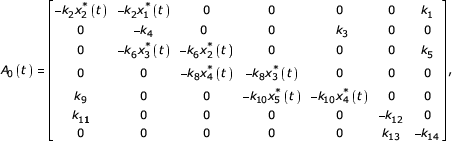The matrix B0 (t) = {Bi,j} where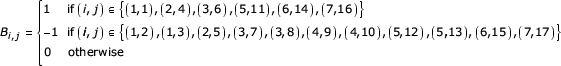Similarly, the matrix C0 (t) = {Ci,j} where all coefficients are zero except for the following: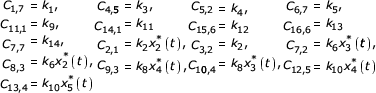Finally, D0 = 0 and the perturbation structure is given by

Δ = diag {δ12234566889101011121314}

Note that since the nominal trajectory is periodic, the matrix functions in the nominal description are also periodic. Note also that in the uncertainty matrix, Δ, some uncertainties are repeated (δ268 and δ10) while δ7 is missing.

The system was then discretized and lifted following the procedure outlined above. A comparison of the system response for each of the approximations at the nominal parameter values is given in Fig. 4B.

For the sampling time we tried various values of n but found negligible differences for values above eight. Finally, we computed the bounds on the SSV. Once again, we found that the values of these two bounds were not affected much by the sampling frequency provided that n is greater than eight. The upper bound was successfully computed using . The maximum over all frequencies is approximately 12.06. However, the high dimension of system causes convergence problem during the computation of μlowerusing this package. To obtain an acceptable lower bound, we calculate the spectral radius at each frequency. This gives us a lower bound for μlower. The plot of the bounds for the SSV when n = 16 is shown in Fig. 6. We can use μlowerto obtain a conservative region for robust stability. The highest value over all frequencies for μloweris approximately 2.636.

## Discussion

Recent years has seen an appreciation that key cellular properties are robust to variations in individual parameter values. Based on the topology of many of these networks, this should not be surprising. Feedback – both negative and positive – control systems are ubiquitous in most biological networks  and one of the reasons for using feedback is that it reduces sensitivity of a system's behaviour to its parameter values.

In modelling biological networks, it is important that this robustness also be in evidence. The particular behaviour being characterized by the model should not rely on precise values of the model's parameters – for example, reaction rate constants or protein concentrations. In particular, a precise measurement of these constants is difficult whereas protein concentrations will vary from one cell to another or throughout the lifetime of any individual. Deviations from the nominal model parameter values should not result in a loss of the network's performance; thus, parameter sensitivity can be used to validate mathematical models of biochemical system. That is, the more insensitive the system response is to the accuracy of the parameter, the more faith we should have in the model .

In looking at certain classes of behaviour, where qualitative changes in the stability of the system are possible, bifurcation diagrams provide an elegant means of evaluating robustness. For example, in evaluating the robustness of the model of Laub and Loomis, of primary importance is determining whether the oscillatory behaviour will persist if the parameter values are altered. This qualitative difference in performance – from limit cycle oscillations to constant steady states – can be quantified and compared across parameters or from one model to another. Once the robustness of the oscillatory behaviour is established, further investigations of the robustness of some of the oscillatory features, for example frequency and amplitude can further be evaluated.

From the bifurcation diagrams obtained for each of the fourteen parameters, we know that oscillations exist only in a limited range around the nominal value. We find the system to be quite sensitive to variations in k2, k4, k10 and k14 and mostly insensitive to the others. Single-parameter bifurcation analysis also shows that the amplitude of the oscillation is greatly affected by the variation of 9 parameters (k1, k2, k4, k6, k7, k10, k11, k12, and k14).

Based on the SSV stability to interpret multiple parameter sensitivity, we can conclude that robust stability of the periodic orbits will be maintained, provided that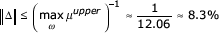Since the uncertainty matrix consists only of diagonal entries, this bound applies to each of the individual parameters. Thus, we can guarantee that the system will be robustly stable provided that no single parameter differs more than 8.3% from its nominal value.

In our analysis we found a large gap between μupperand our lower bound for μ. As we later show, for this system the upper bound is fairly tight, as we are able to obtain a destabilizing perturbation of size 9%. For general biological models, a robustness measure based on the upper bound μuppermay also be more appropriate. Robustness bounds for systems in which arbitrarily slowly-time-varying parameter values are allowed are known . For these systems it has been shown that the bounds converge as the time-variations approach zero to the upper bound μupper. Since many of the parameters in models of biochemical networks represent features that will vary over time, such as enzyme concentrations, this number may therefore be more indicative of the model's true robustness.

The ability to consider the effect of time-variations on the robustness of the system is one great advantage of the SSV over other methodologies. One drawback of the SSV approach compared to the bifurcation theory is that it does not provide the precise combination of parameters that destabilizes the system – only its size. Also, since the upper bound is only sufficient to guarantee robustness, this number may, in general, give an overly conservative notion of robustness.

It must be emphasized that the SSV approach denoted here is based on the linearized model of the system. For some classes of systems this linearization may not be possible – in this case, the linear SSV approach documented here will not be applicable. However, for most models used to describe biochemical reactions, this should not be a problem.

Because we are concentrating exclusively on the local stability of the linearized model, important parameters of the oscillatory behaviour such as robustness of the frequency and amplitudes of oscillation are not evaluated. Also, the effect of parameter variations on the equilibrium orbit are omitted. In particular, varying the kinetic parameters will change the behaviour of the system in two different ways: the equilibrium periodic orbit will change and the stability of deviations about this orbit will also change. The SSV allows one to quantify the robustness of the second of these two effects. It does not say anything directly regarding the effect of parameter variations on the equilibrium periodic orbit. One way of bounding the effect of these parameter changes is to write the original differential equation as(t) = f(x,k)

where k = k0 + δ is the set of kinetic parameters with nominal values k0. If the nominal periodic orbit (when δ = 0) is given by

x*(t) = x(t) - xδ (t)δ(t) = A(t)xδ (t) + v

where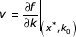δ is a constant vector that includes the effect of this parametric uncertainty. Thus, the system can be considered as being perturbed by a constant input signal v. Provided that the homogeneous system is exponentially stable (and this is guaranteed by the existence of a stable limit cycle) and that v is not "too large", the perturbed system's state will remain in a neighbourhood of the origin if the f(x,p) in the original equation is reasonably well behaved in k. Detailed bounds and conditions on f are given in Theorem 5.1 of , though it should be emphasized that these bounds tend to be overly conservative in practice.

To illustrate the local nature of the SSV analysis for this system, we perturbed the system parameters by varying amounts. The particular parameters were either increased or decreased so as to bring them closer to the Hopf bifurcation. For example, the nominal value of k1 is closer to k1 than to1 so that we reduced k1 whereas k4 is closer to4 than to k4 so we increased k4. In Fig. 7 we show the response of these systems to changes of 7% and 9% both for the linearized system – where the linearized response has been superimposed on the nominal limit cycle (Fig. 7A) and the original non-linear system (Fig. 7B). For the smaller value, the linearized response is stable and we see that, after a transient, the response settles to the nominal limit cycle. We also see this same behaviour in the response of the non-linear system with this level of parameter perturbation. For a 9% change in the parameters, however, the linearized system is unstable. We see this as a deviation from the nominal limit cycle. In the non-linear system's response, this translates into an end to the stable limit cycle. The response does not "blow up" but instead settles into a fixed point.

This example illustrates how a robustness analysis of the linearized system can be used to deduce the robustness of the original non-linear system, as it shows that when the linearized system is unstable, the desired behaviour of the non-linear system will no longer be present. This example also points out the fact that the upper bound μupper 8.3% is not overly conservative for this system as we were able to produce a destabilizing perturbation of size 9%.

Finally, we note that multiparametric robustness analysis considered here is based on local properties of the dynamical system, since we are evaluating the robustness of the linearized model. Extensions to the non-linear model are the subject of active investigation .

However, it is by combining the robustness analysis of both single and multiple parameters, we can obtain a more thorough understanding of the region of stability of the periodic solution in the high dimensional parameter space and use this to improve upon the model. In this particular example, we find that the system's robustness is governed by several "robustness limiting" parameters, k2, k4, k10 and k14.

## Conclusions

Determining the robustness of mathematical models of biological systems is important for several reasons. First, there is growing evidence that many aspects of the networks being modelled have evolved in such a way so that they are robust as this allows them to tolerate natural variations in the environment. Thus, faithful models should replicate this robustness. Second, robustness of the models provide a means of validating model quality since the performance of the models should not rely on precisely tuned parameter values that are impossible – or at best – difficult to measure exactly.

In this paper, we illustrated the use of two tools developed in dynamical systems theory and control engineering to assess robustness quantitatively. For an example, we considered an oscillatory molecular network model due to Laub and Loomis that aims at describing oscillatory behaviour in cAMP signalling observed in the social amoeba D. discoideum. This behaviour appears as a stable limit cycle of the equations describing the model. We have evaluated the degree to which this limit cycle is robust to variations in all the system parameters.

The robustness of the oscillatory behaviour to single parameter variations was quantified using bifurcation analysis. Using the bifurcation analysis software tool AUTO we determined that single parameter changes as small as 20% from the nominal value can cause the limit cycle to disappear and a stable equilibrium to appear. In addition to the stability robustness, AUTO is also able to evaluate the sensitivity of the amplitude of the oscillation to these parameter changes.

To investigate the robustness of the model to simultaneous changes in parameter values, the structured singular value (SSV) analysis tool was used. Once the system was in the correct framework for SSV analysis, we were able to determine that the system can only tolerate very small changes in the parameter values – in the order of 8% – if we allow these parameters to vary with time arbitrarily slowly.

Finally, it is important to note that to understand completely the robustness properties of a model, it is appropriate to combine single and multiple parameter sensitivity analyses.

## References

1. 1.

Barkai N, Leibler S: Robustness in simple biochemical networks. Nature 1997, 387: 913–917. 10.1038/43199

2. 2.

Alon U, Surette MG, Barkai N, Leibler S: Robustness in bacterial chemotaxis. Nature 1999, 397: 168–171. 10.1038/16483

3. 3.

von Dassow G, Meir E, Munro EM, Odell GM: The segment polarity network is a robust developmental module. Nature 2000, 406: 188–192. 10.1038/35018085

4. 4.

Meir E, von Dassow G, Munro E, Odell GM: Robustness, flexibility, and the role of lateral inhibition in the neurogenic network. Curr Biol 2002, 12: 778–786. 10.1016/S0960-9822(02)00839-4

5. 5.

Savageau MA: Parameter sensitivity as a criterion for evaluating and comparing the performance of biochemical systems. Nature 1971, 229: 542–544.

6. 6.

Morohashi M, Winn AE, Borisuk MT, Bolouri H, Doyle J, Kitano H: Robustness as a measure of plausibility in models of biochemical networks. J Theor Biol 2002, 216: 19–30. 10.1006/jtbi.2002.2537

7. 7.

Alves R, Savageau MA: Extending the method of mathematically controlled comparison to include numerical comparisons. Bioinformatics 2000, 16: 786–798. 10.1093/bioinformatics/16.9.786

8. 8.

Laub MT, Loomis WF: A molecular network that produces spontaneous oscillations in excitable cells of Dictyostelium . Mol Biol Cell 1998, 9: 3521–3532.

9. 9.

Liu JS: Monte Carlo strategies in scientific computing New York: Springer 2001.

10. 10.

Zhou K, Doyle JC, Glover K: Robust and optimal control Englewood Cliffs, NJ: Prentice-Hall 1996.

11. 11.

Strogatz SH: Nonlinear dynamics and chaos Cambridge: Perseus 1994.

12. 12.

Borisuk MT, Tyson JJ: Bifurcation analysis of a model of mitotic control in frog eggs. Journal of Theoretical Biology 1998, 195: 69–85. 10.1006/jtbi.1998.0781

13. 13.

Enns-Ruttan JS, Miura RM: Spontaneous secondary spiking in excitable cells. Journal of Theoretical Biology 2000, 205: 181–199. 10.1006/jtbi.2000.2056

14. 14.

Doedel E, Champneys AR, Fairgrieve TF, Kuznetsov YA, Sandstede B, Wang XJ: AUTO 97: Continuation and bifurcation software for ordinary differential equations Montréal, Canada: Publisher 1997.

15. 15.

Doyle J: Analysis of feedback-systems with structured uncertainties. IEE Proceedings-D Control Theory and Applications 1982, 129: 242–250.

16. 16.

Zames G: On input-output stability of time-varying nonlinear feedback systems, I: Conditions derived using concepts of loop gain conicity and positivity. IEEE Transactions on Automatic Control 1966, AC11: 228–238.

17. 17.

Khalil HK: Nonlinear systems 3 Edition Englewood Cliffs, NJ: Prentice Hall 2002.

18. 18.

Chen T, Francis BA: Optimal sampled-data control systems London: Springer-Verlag 1995.

19. 19.

Rugh WJ: Linear system theory Englewood Cliffs, NJ: Prentice-Hall 1996.

20. 20.

Qiu L, Bernhardsson B, Rantzer A, Davison EJ, Young PM, Doyle JC: A formula for computation of the real stability radius. Automatica 1995, 31: 879–890. 10.1016/0005-1098(95)00024-Q

21. 21.

Braatz RP, Young PM, Doyle JC, Morari M: Computational-complexity of μ-calculation. IEEE Transactions on Automatic Control 1994, 39: 1000–1002. 10.1109/9.284879

22. 22.

Balas GJ, Doyle JC, Glover K, Packard A, Smith R: μ-analysis and synthesis toolbox (Mu-Tools). Automatica 1994, 30: 733–735. 10.1016/0005-1098(94)90164-3

23. 23.

Gerisch G, Wick U: Intracellular oscillations and release of cyclic AMP from Dictyostelium cells. Biochem Biophys Res Commun 1975, 65: 364–370.

24. 24.

Freeman M: Feedback control of intercellular signalling in development. Nature 2000, 408: 313–319. 10.1038/35042500

25. 25.

Haefner JW: Modeling biological systems: Principles and applications New York: Chapman and Hall 1996.

26. 26.

Jönsson U, Rantzer A: Systems with uncertain parameters – time-variations with bounded derivatives. Internat J Robust Nonlinear Control 1996, 6: 969–982. Publisher Full Text 10.1002/(SICI)1099-1239(199611)6:9/10%3C969::AID-RNC262%3E3.3.CO;2-R

27. 27.

Parrilo PA: Structured semidefinite programs and semialgebraic geometry methods in robustness and optimization Pasadena, CA: California Institute of Technology 2000.

## Acknowledgements

We thank J. Krishnan and W.J. Rugh for useful comments on the manuscript. This work was supported in part by the Whitaker Foundation and the National Science Foundation's Biocomplexity program, through grant number DMS-0083500.

## Author information

Authors

### Corresponding author

Correspondence to Pablo A Iglesias.

### Authors' contributions

LM carried out the computational studies and analysis. PAI conceived of the study and participated in its design and coordination. All authors read and approved the final manuscript.

## Authors’ original submitted files for images

Below are the links to the authors’ original submitted files for images.

## Rights and permissions

Reprints and Permissions

Ma, L., Iglesias, P.A. Quantifying robustness of biochemical network models. BMC Bioinformatics 3, 38 (2002). https://0-doi-org.brum.beds.ac.uk/10.1186/1471-2105-3-38